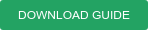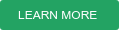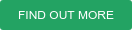With the near-constant advances in smart technology and science, there is an increased demand on satellites. To improve satellite capabilities and meet the needs of the market, organisations are commercialising multimillion-dollar equipment and developing more efficient testing techniques for space technology.

GEO, MEO and LEO satellites navigate the earth surrounding space on different altitudes. All of them use thrusters to make small adjustments to their position and altitude. While thrusters operate with different types of propellant material, here we focus on electric propulsion (EP) — and more specifically ion thrusters.

Xenon has long been the choice for EP. However, the high price of xenon and the increase in demand is pushing organisations to find a cheaper alternative. This has led to krypton becoming the propellant of choice for several space missions. In the following, we share a general guide for building vacuum systems for thruster testing chambers.

As we’ve mentioned in our previous blog on vacuum technology for space simulation chambers, a combination of a Gifford McMahon type cryocooler and a condensing plate (cryopanel) are used to pump xenon during the testing process of the thruster. Krypton gas is condensed in the same way. However, different criteria must be considered depending on the nature of the propellant being used.

## 1. PART A

Before determining the number of cryopanels needed to achieve the desired pumping speed, we must first determine the type of cold head most suitable for the application i.e. based on the process parameters.

The cold head is determined by:

1. Type of propellant gas: Krypton (Kr), Xenon (Xe)
2. Process pressure is usually taken in the range of 5e-6 mbar to 5e-5 mbar.

As a rule of thumb, the chosen condensation temperature for krypton shall lead to a saturation vapour pressure of one order of magnitude lower than the required pressure. This is to account for any increase of temperature after condensation that might lead to an increase of pressure.

Thus, we chose T= 33K as condensation temperature for krypton and T = 45 K for xenon.  This keeps us in the plateau region of the maximum pumping speed per surface area (see figure 1b)Figure 1: a) saturation vapour pressure in function of temperature. b) Max. pumping speed per panel surface area for Kr and Xe as a function of panel temperature at process pressure 1e-3 Pa.

1. In general, single-stage cold heads are used for this type of application in combination with a cryopanel. Figure 2 shows the cooling performance of the pneumatic cold head CP 140i and the mechanically driven cold head CP 250 MDi — both made by Leybold.

N.B: the cooling capacity represents the maximum heat load in Watt that can be applied on the panel to maintain the temperature needed.

##Figure 2: Cooling performance of the Leybold cold heads CP 250MDi and CP 140i as a function of temperature.

As an example, we chose the Leybold cold head CP 250 MDi, which has a cooling capacity of 83W at 33K (for Kr condensation) and 122 W at 45 K (for Xe condensation).

B. Having chosen a suitable cold head and assessing its cooling capacity, we can now calculate the maximum allowable diameter for the cryopanel that we can attach to the cold head.

To make this calculation, We consider heat transfer via radiation as the only form of heat transfer; this is because the process happens at high vacuum.

This estimation is made taking into account the following safety margins:

1. The maximum heat load is equal to cooling capacity at T= 33K and T= 45K
2. Emission coefficient of the chamber walls equal to 1; εw= 1 (black body radiation). This is to consider that there might be sputtering and/or erosion of the chamber walls after an extended period of the thruster testing. This will affect the emissivity of the chamber walls. The original emissivity is between 0.4 - 0.7 for clean stainless-steel walls. Depending on the treatment, it can also be lower than the mentioned values.
3. Emission coefficient of the cryopanels (Ni plate copper plates εk ~ 0.1) is taken equal to 0.7 to account for thick layers of ice that form on the surface of the panels after several hours of pumping.Where σ is defined as the Stefan-Botlzmann constant, this calculation can be extrapolated as:

Q = f*εk* Ak*σ* (Tw4-Tk4)

f = 1/(1+ εk* Ak* (1/εw -1)/Aw )

A=Aw

f = 1/(1+ εk*  (1/εw -1))

Ak= Q/ (f*εk*σ* (Tw4-Tk4))

We consider Aw (area of the wall) = Ak (area of the cryopanel) as two parallel plates (maximum heat load) with a view factor equal to 1. Tk = 33K or 45 K and Tw = 298 K.

Using the above mentioned equation and values, we calculate the maximum allowable panel diameter depending on the cold head and the gas to be pumped. We also provide the limit that we need to adhere to insure high and stable pumping speed and process pressure.

Once the diameter of the panel is chosen, we can then calculate the number panels for the total flow of Krypton and Xenon

## 2. PART B

To calculate the number of cold heads and cryopanels needed, we first calculate:

1. Pumping speed per surface area — SA = SQRT (kB*10*T/(2*M*π))
2. Theoretical pumping speed per panel — S= SA *Ak (panel area). Here we only consider the front surface of the panel as the pumping surface.
3. Having SA (Kr) = 6.6 l/s.cm2 and SA (Xe) = 5.44 l/s.cm2 , panels pumping speed can be calculated for each gas as: we estimate a reduction of the pumping speed by 10% as a safety margin making the effective pumping speed equal to Seff = S*0.9 = SA *Ak *0.9
4. Now we consider the flow of each gas at a given time: at a certain gas flow dqpv/dt [Pa.l/s] the needed pumping speed Stot is calculated depending on the process pressure P as follows Stot = dqpv/dt / P

Having the total needed pumping speed, the needed number of panels can be calculated by dividing Stot by the effective pumping speed of the panel.

The number of needed panels is therefore; N = Stot / Seff

## THE NEXT STEP IN SPACE AND THRUSTER TECHNOLOGY

Without fit-for-purpose testing chambers and vacuum simulations, it would be impossible to improve the capabilities of space and satellite technology. In this guide, we’ve shared calculations that will support you in building testing chambers for thrusters that use either Krypton or Xenon gas.

We’ve written an eBook that explores more aspects of vacuum simulation. In it, we unpack:

• The technical and theoretical background of vacuum simulation
• The implications of simulation for different pressure ranges and products
• How to overcome vacuum simulation complexities
• The different bespoke simulation tools available

Click the button below to get your free copy of An Introduction to Vacuum Simulation.### Filed Under:

Vacuum Simulation

BESPOKE VACUUM SYSTEM DESIGN AND RECOMMENDATIONS